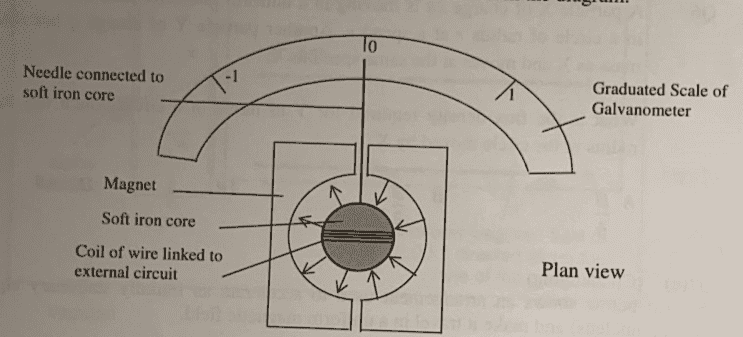# Shape of graph of torque vs angular displacement of galvanometer

songoku
Homework Statement:
The diagram below shows galvanometer. A coil of wire is wound around a circular iron core which is placed between two magnets with circular surface such that the magnetic field (indicated by arrows) on the surface of iron core is directed perpendicularly onto the surface and of the same magnitude across the surface of iron core as shown in diagram.

When a constant current flows in the coil, the needle will be deflected to angle ##\theta## from the vertical direction. Sketch a graph to show the variation of torque ##\tau## on the soft iron core due to magnetic force acting on the coil with the angular displacement ##\theta## of the needle as the needle rotates from zero to ##\theta_0##. Explain
Relevant Equations:
##\tau## = F . dI know the torque will be zero when the deflection is zero and will be maximum when the angular displacement is θ0 but how to determine the exact shape of the graph?

Thanks

Homework Helper
Gold Member
2022 Award
The question asks for the relationship between the torque and the deflection. Note that this has nothing to do with the current or the magnets. You could throw those away and twist the coil by hand and get the same result.
So what does control the relationship?

•songoku
songoku
The question asks for the relationship between the torque and the deflection. Note that this has nothing to do with the current or the magnets.
I thought the value of torque acting on the needle is somewhat related to current flowing through the galvanometer, maybe something like F = BIL and τ = F.d so the magnitude of current also affects the deflection.

If torque has nothing to do with current of magnetic field, how can torque on the needle produced?

You could throw those away and twist the coil by hand and get the same result. So what does control the relationship?
Maybe magnitude of force applied by hand?

Thanks

Homework Helper
Gold Member
2022 Award
I thought the value of torque acting on the needle is somewhat related to current flowing through the galvanometer, maybe something like F = BIL and τ = F.d so the magnitude of current also affects the deflection.

If torque has nothing to do with current of magnetic field, how can torque on the needle produced?

Maybe magnitude of force applied by hand?

Thanks
The current creates the torque, and the torque creates the deflection. A given value of torque, no matter how provided, results in a certain deflection.
What determines how much deflection results from a given torque?
Hint: if the current is switched off what happens to the needle? Why does that happen?

•songoku
songoku
The current creates the torque, and the torque creates the deflection. A given value of torque, no matter how provided, results in a certain deflection.
What determines how much deflection results from a given torque?
Hint: if the current is switched off what happens to the needle? Why does that happen?
If the current is switched off, the needle will return to original position because there is restoring torque from spring. So it means that the deflection will be related to torque by spring.

Formula of restoring torque is: ##\tau = k.\theta## so the shape of the graph will be linear line similar to y = mx + c.

Am I correct? Thanks

•Tom.G and Lnewqban
Homework Helper
Gold Member
2022 Award
If the current is switched off, the needle will return to original position because there is restoring torque from spring. So it means that the deflection will be related to torque by spring.

Formula of restoring torque is: ##\tau = k.\theta## so the shape of the graph will be linear line similar to y = mx + c.

Am I correct? Thanks
Yes.

•songoku
songoku
Thank you very much haruspex